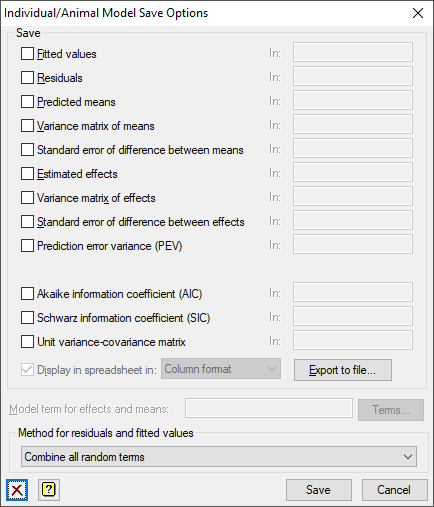1. Home
2. Individual/Animal Model Save Options

# Individual/Animal Model Save Options

Use this to save results from a fitting an individual/animal model in Genstat data structures.

1. After selecting the appropriate boxes, type the identifiers of the data structures into the corresponding In: fields.## Save

 Fitted values Variate Fitted values from the analysis Residuals Variate Residuals from the analysis Predicted means Table Predicted means for the specified term Variance matrix of means Symmetric matrix Variance-covariance matrix for the predicted means Standard error of difference between means Symmetric matrix Standard errors of differences between the predicted means Estimated effects Table Estimated regression coefficients for the specified term Variance matrix of effects Symmetric matrix Variance-covariance matrix for the estimated effects Standard error of difference between effects Symmetric matrix Standard errors of differences between the estimated parameters of each term Predicted error variance Diagonal matrix Predicted error variance for the specified term Akaike information coefficient (AIC) Scalar Akaike information coefficient to assess the random model Schwarz information coefficient (SIC) Scalar Schwarz information coefficient to assess the random model Unit variance-covariance matrix Symmetrical matrix Matrix giving the fitted variance-covariance relationship between all the units. Note: this may be very large. This uses the random and residual terms, but not spline terms. It cannot be formed if the model contains sparse inverse covariance matrices.

Select this to display the results in a new spreadsheet window.
The format of the table of Predicted means and Estimated effects is controlled by the Format dropdown list.

 Page format Each table is displayed on a single page in a separate spreadsheet. The last specified classifying factor indexes the columns in the spreadsheet. Column format The tables occupy a single column but multiple tables can be put in a single spreadsheet. This is the default for a table with a single classifying factor.

## Model terms for effects and means

Specifies the model term for which tables of estimated effects and predicted means are to be saved. The string ‘Constant’ may be used to save results for the constant term. If tables of effects or means are required for more than one model term, this menu should be invoked once for each term, changing the specification of the model term each time.

## Method for residuals and fitted values

Residuals can be formed from either all random terms, or just the final residual stratum (default).

Updated on October 29, 2020